## 2D Convex Polygon Collision using SAT

#### INTRODUCTION

SAT or Separating Axis Theorem is the de facto standard in 2d polygon collision. Most 2d (and 3d) physics engine use SAT in one way or another to collide shapes very fast. SAT is useful for games in general because the algorithm itself is very fast when there are not a lot of colliding objects. I wrote this article because someone asked me to and most articles about the subject are either too vague or too mathematically muddled to be of use for use mere humans. However, if you go out of your way and analyze the algorithm, SAT is very simple.

Pros:

• SAT is an "early-out" algorithm.
• SAT can be optimized to only check half the edges in special cases
• SAT results are still useful even without normalized axis-testing (If you only want to test intersections)
• SAT is very fast for games where there isn’t a lot off colliding objects.
• SAT can be used to approximate the shapes of your sprites, eliminating the need to do a very slow pixel perfect collision.

Cons:

• SAT is limited to convex polygons.
• SAT is not very fast for systems where there are more than 95% of colliding bodies. But what’s the alternative?

A "Convex Polygon" is A polygon such that no side extended cuts any other side or vertex; it can be cut by a line in at most two points.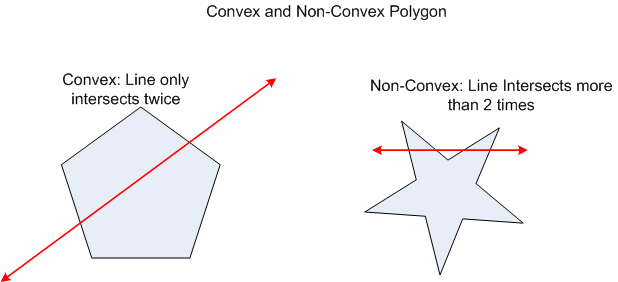#### DEFINITION

SAT mantra: "If two convex objects are not penetrating(intersecting), there exist and axis in which the projection of the objects will not overlap"

Okay, that’s too nerdy for us programmers (well, we are all essentially nerds in some sense. We just don’t want to admit it) so I’ll explain it in a more earthly way.

All it says is that when you have 2 convex polygons in 2d space (ie. screen), they are not intersecting if and only if, you can find an axis (vector) that separates them. The "projection of objects" are the projection of each of the vertex of each polygon onto the axis.

#### MATHEMATICS NEEDED

1. Normal to a line /edge (a vector perpendicular to a line)

Given a line (x2-x1,y2-y1)…

The normals to that line are:

```N( -(y2-y1),(x2-x1) )
or
N( (y2-y1),-(x2-x1) )```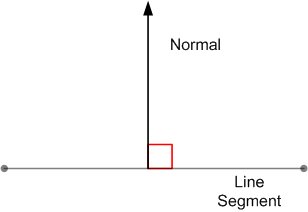Code(Notice that we can choose either the right or left normals):

```function get_2dnormal ( byval x1 as single, byval y1 as single,_
byval x2 as single, byval y2 as single,_
byval s as integer) as vector2d
dim normal as vector2d
if s then
normal.x = -(y2-y1)
normal.y =  (x2-x1)
else
normal.x =  (y2-y1)
normal.y = -(x2-x1)
end if
normalize (normal)
return normal
end function```

2. Vector Normalization (make the vector’s length = 1)

Length = sqrt(v.x^2 + v.y^2)

v.x = v.x/length

v.y = v.y/length

Code:

```sub normalize (byref v as vector2d)
dim leng as single
leng = sqr(v.x * v.x + v.y * v.y )
v.x = v.x / leng
v.y = v.y / leng
end sub```

3. Dot product or inner product (returns a scalar(real number) value that is equal to the length of projection of vector a onto vector b)

`Vector_dot = ( v.x *u.x  + v.y *u.y )`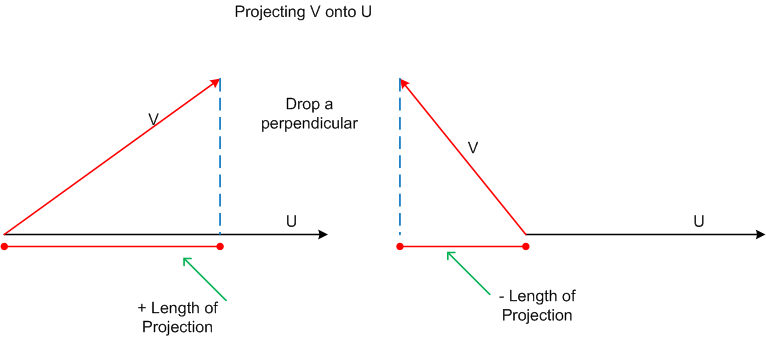Code:

```function dot(byval a as vector2d,byval  b as vector2d) as Single
return (a.x*b.x + a.y*b.y )
end function```

Here’s a visual demo to the dot product in action:

dot_project.zip

That’s it. Just three (Actually, if you only need to check for intersection, you don’t need to normalize your axis so you only need two.).

#### ALGORITHM

So how do you go about coding SAT? I must admit that when I first decided to code my SAT implementation (back in 2k8), It took me a while to figure out how to even start. In fact I didn’t believe the algorithm could work. Only after drawing things on paper did I admit that it works.

SAT tests for an instance of an axis of separation by this general algorithm:

1. For each of the edge of both polygons…
2. Get the axis to test (the current edge normal)
3. Project(dot product) all the vertices of polygon 1 to that axis and get the minimum and maxumum projection values.
4. Project(dot product) all the vertices of polygon 2 to that same axis and get the minimum and maximum projection values.
5. If the projections does not overlap, then we can never collide so return a false value and exit. This is the "early-out" system I was talking about than makes SAT very fast for non-colliding object. Note that this is a 1-d overlap check.

You will notice that you need to check all the axis-normals for us to be able to be certain that the two polygons collide. That is why it’s not optimized for colliding objects.

So how do we project a vertex to an axis? Simply use the dot product.

Here we have a polygon and an axis: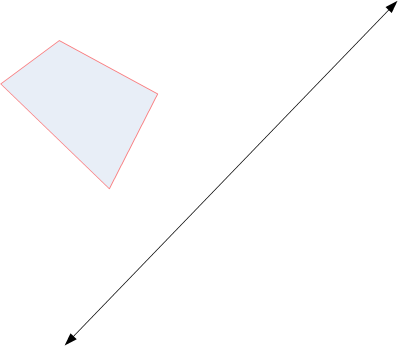Projecting its vertices…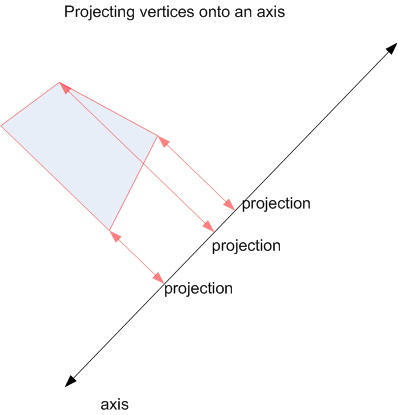When we project all the vertices of a polygon on an axis we get projection(scalar) values. The number returned by the dot poduct corresponds to the distance of the projection of a particular vertex onto the axis. Now for triangles we get 3 projection values, 6 for hexagons and so on but we only need two values to check for overlaps. We only need the minimum and maxumum projections.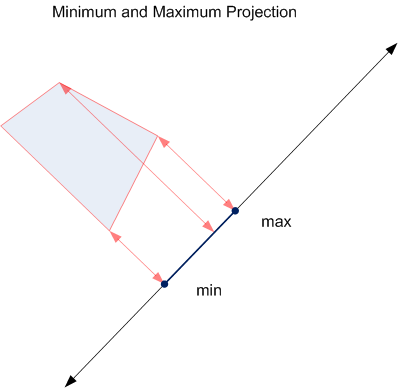Code for polygon vertex to axis projection:

```''**********************************************************************************
''
''	min, max overlap stuff
''
''**********************************************************************************
type Tprojection
min			as single
max			as single
end type
''**********************************************************************************
''
''	meat of the SAT algo
''  projects each vertex of the poly to
''	the axis and gets the min and max 1d values
''
''**********************************************************************************
function Tpolygon.project(byval _axis as vector2d) as Tprojection
dim as Tprojection proj
dim as single min, max
dim as single p
'' get initial projection on first vertex
'' and set min and max values to initial projection value
p = dot(verts_2(0), _axis)
min = p
max = p
'' loop through all the verts of the poly
'' and find out if new projections of vertex
'' is the new min or max as we need only the
'' min and max points to test for 1d intesection
for i as integer = 1 to numverts-1
p = dot(verts_2(i), _axis)
if (p < min) then min = p
if (p > max) then max = p
next i
'' copy and return
proj.min = min
proj.max = max
return proj
end function ```

How is this useful in testing if two polygons intersect? Well, by testing whether the min and max projections of two polygons on an axis does not overlap, we can be sure that they do not intersect. Let’s look at this diagram: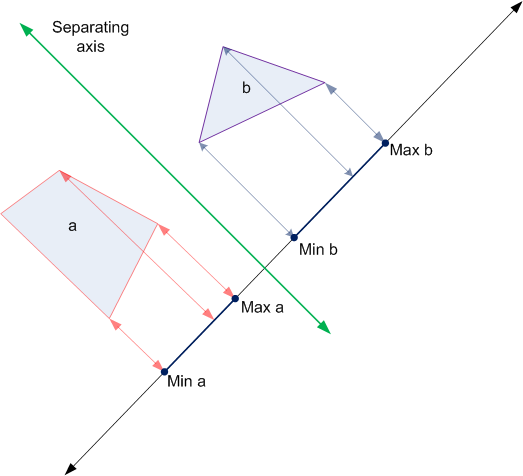These two polygons do not intersect because their min and max values do not overlap on the axis. I also pointed out the separating axis (green) that separates them. Now for another diagram…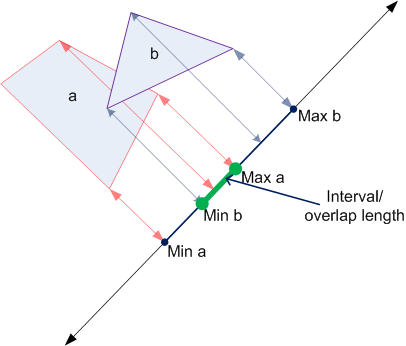In the above diagram, the two polygons are intersecting. Notice that their projections overlap (minB and maxA). So we can safely conclude from that diagram that the two polygons are colliding on that particular axis. However, we can never be sure of an intersection until we check all the relevant axis. So what are the "relevant axis" to check both polygons for? It turns out that we can use both polygons’ edge normals for this.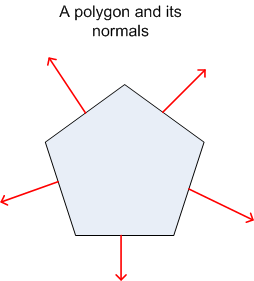Those red arrows are the normals.

Projecting polygon A onto all the axes of polygon B…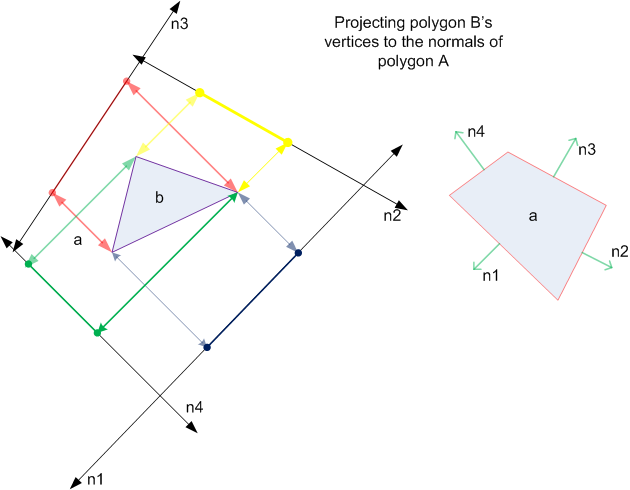Pseudocode to do the SAT between A and B:

• For all of A’s axes(edge normals)
• Project A
• Project B
• If projections does not overlap return false.
• Loop
• Do the same for B’s axes
• If we ever get here, Poly A and Poly B are intesecting. Return true

Code for Poly to poly check:

```''**********************************************************************************
''
''	checks whether poly1 and poly2 collides using SAT
''  use this if you just need to do simple intersection test
''
''**********************************************************************************
function poly_collide(byref p1 as Tpolygon, byref p2 as Tpolygon) as integer
dim as Tprojection proj1, proj2
dim as vector2d axis
dim as single d1, d2
'' project all the verts of the poly to each axis (normal)
'' of the poly we are testing and find out if the projections
'' overlap (ie: length if proj1 and proj2 are intersecting).
'' if they are intersecting, there is an axis (line perpendicular
'' to the axis tested or the "edge" of the poly where the normal connects)
'' that separates the two polygons so we do an early out from the function.
'' polygon1
for i as integer = 0 to p1.numverts - 1
axis = p1.normals(i)
proj1 = p1.project(axis)
proj2 = p2.project(axis)
d1 = (proj1.min - proj2.max)
d2 = (proj2.min - proj1.max)
if ((d1 > 0) or (d2 > 0)) then
'' there's a separatng axis so get out early
return  false
end if
next i
'' polygon2
for i as integer = 0 to p2.numverts - 1
axis = p2.normals(i)
proj1 = p1.project(axis)
proj2 = p2.project(axis)
d1 = (proj1.min - proj2.max)
d2 = (proj2.min - proj1.max)
if ((d1 > 0) or (d2 > 0)) then
'' there's a separatng axis so get out early
return  false
end if
next i
'' no separating axis found so p1 and p2 are colliding
return true
end function```

Pretty easy and straightforward right?

#### CALCULATING THE MTV

MTV or Minimum Translation Vector is the vector needed to push the objects away from each other or the "overlap length" of the two polygons.

To calculate the MTV is pretty straightforward considering that we have already done much of the work to calculate it. It turns out that the MTV is the axis that we check for projections itself and the magnitude is the overlap of the least 1-d length.

Ie:

• Project all vertices to axis
• Get interval
• If interval > 0, exit because there’s no overlap
• If interval is < min_interval then
• min_interval = interval
• min_translation_vector = axis
• end if
• If we can’t find a separating axis….
• Mtv = vector(min_translation_vector, min_interval)Pretty easy. However there are a few things to do in order to make it work as expected. One is to find the new direction of the MTV and the point of contact then handle vertex to edge collisions, which I have done on this code snippet:

Code:

```''**********************************************************************************
''
''	checks whether poly1 and poly2 collides using SAT + MTV
''  use this if you want real physics
''
''  velocity is the velocity of polygon 1
''  add a simple time value and you have a swept test
''
''**********************************************************************************
function poly_collide_MTV(byref p1 as Tpolygon, byref p2 as Tpolygon, byref mtv as vector2d, byref velocity as vector2d) as integer
dim as Tprojection proj1, proj2
dim as vector2d axis
dim as single d1, d2
dim as single magnitude, overlap
dim as single interval_distance
'' project all the verts of the poly to each axis (normal)
'' of the poly we are testing and find out if the projections
'' overlap (ie: length if proj1 and proj2 are intersecting).
'' if they are intersecting, there is an axis (line perpendicular
'' to the axis tested or the "edge" of the poly where the normal connects)
'' that separates the two polygons so we do an early out from the function.
'' polygon1
magnitude = 9999999
'' uber large numbah
for i as integer = 0 to p1.numverts - 1
axis = p1.normals(i)
'' get axis to test
proj1 = p1.project(axis)
'' project vertices
proj2 = p2.project(axis)
'' get 1-d interval distance
interval_distance = get_interval_distance(proj1, proj2)
if (interval_distance > 0) then
'' Since there's no overlap, there's a separating axis so get out early
return false
endif
'' project velocity of polygon 1 to axis
dim as single v_proj = dot(axis, velocity)
'' get the projection of polygon a during movement
if (v_proj < 0) then
'' left
proj1.min += v_proj
else
'' right
proj1.max += v_proj
endif
'' get new interval distance
'' ie. (p1 + velocity) vs (non-moving p2)
interval_distance = get_interval_distance(proj1, proj2)
'' get the absolute value of the overlap
overlap = abs(interval_distance)
'' overlap is less than last overlap
'' so get the MTV
'' MTV = axis
'' magnitude = minimum overlap
if (overlap < magnitude) then
magnitude = overlap
mtv.x = axis.x
mtv.y = axis.y
'' project distance of p1 to p2 onto axis
'' if it's on the left, do nothing as
'' we are using the left-hand normals
'' negate translation vector if projection is on the right side
dim as vector2d vd = type(p1.x - p2.x, p1.y - p2.y)
if ( dot(vd, axis) > 0 ) then
mtv.x = -axis.x
mtv.y = -axis.y
endif
endif
next i
'' polygon2
for i as integer = 0 to p2.numverts - 1
axis = p2.normals(i)
proj1 = p1.project(axis)
proj2 = p2.project(axis)
'' get 1-d interval distance
interval_distance = get_interval_distance(proj1, proj2)
if (interval_distance > 0) then
'' Since there's no overlap, there's a separating axis so get out early
return false
endif
'' project velocity of polygon 1 to axis
dim as single v_proj = dot(axis, velocity)
'' get the projection of polygon a during movement
if (v_proj < 0) then
'' left
proj1.min += v_proj
else
'' right
proj1.max += v_proj
endif
'' get new interval distance
'' ie. (p1 + velocity) vs (non-moving p2)
interval_distance = get_interval_distance(proj1, proj2)
'' get the absolute value of the overlap
overlap = abs(interval_distance)
'' overlap is less than last overlap
'' so get the MTV
'' MTV = axis
'' magnitude = minimum overlap
if (overlap < magnitude) then
magnitude = overlap
mtv.x = axis.x
mtv.y = axis.y
'' project distance of p1 to p2 onto axis
'' if it's on the left, do nothing as
'' we are using the left-hand normals
'' negate translation vector if projection is on the right side
dim as vector2d vd = type(p1.x - p2.x, p1.y - p2.y)
if ( dot(vd, axis) > 0 ) then
mtv.x = -axis.x
mtv.y = -axis.y
endif
endif
next i
'' if we get to this point, the polygons are intersecting so
'' scale the normalized MTV with the minimum magnitude
mtv.x *= magnitude
mtv.y *= magnitude
'' no separating axis found so p1 and p2 are colliding
return true
end function```

Now all you have to do when colliding is add the MTV to your translation vector and voila! Easy and fast collision.

#### THE SWEPT TEST

A swept test simply put, is a test between two objects where one is moving and the other isn’t. If you have run the demo, you must have noticed that the objects actually collide before being pushed back by the MTV. However for a real physics simulation, the objects should never intersect at all. This is where the swept test comes in.

In order to add a swept test we must add one little test within the collide function. Intead of testing whether we intersect and exiting the test we:

Given two Polygons A and B that are not intersecting…

• For every axis
• Extend the projection of Poly A by adding its velocity projection to its min and max projections.
• Do the interval test as before.
• If they "will" intersect, calculate the MTV and exit.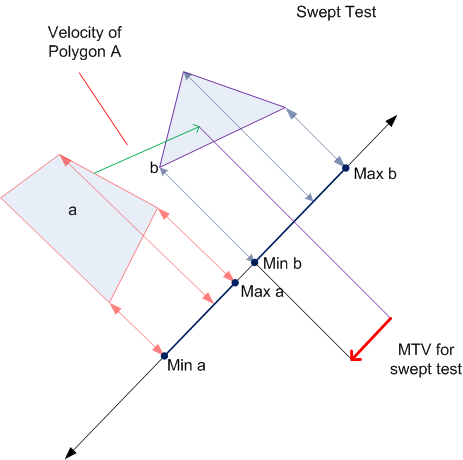#### OPTIMIZATIONS and TIPS

• For regular polygons(equilateral and equiangular) like a rectangle, the test could be reduced by ½ since each edge has a corresponding parallel edge.
• If you only need to test for an intersection, you don’t need to normalize your axes. Therefore eliminating a costly sqrt.
• Precalculate your normals for polygons that never change shape.
• For polygons whos shapes don’t change (like in my example), but needed to be rotated, you can precalculate your normals and rotate them with the same rotation values as your vertices.
• For circles vs. polygons, you will only need to check for 1 axis. You can get that axis from the vector defined by closest vertex to the circle center and do the MTV projection method above. I’ll leave that as an exercise for the reader.

EXAMPLES:

sat_examples.zip

Freebasic:

Example

C++(Fixed point)

Example

#### CONCLUSION

Well, that all for my SAT article. I hope you have enjoyed it. The code in those examples are not very optimized for clarity’s sake so it’s up to the reader to optimize it according to his/her needs. Oh yeah, my drawing sucks!

For questions you can contact me through:

Powered by CMSimple | CMSimple Legal Notices | (X)html | css | Login | Template-Design: Lars Ellmauer | Template-Modified: Imortisoft ISD